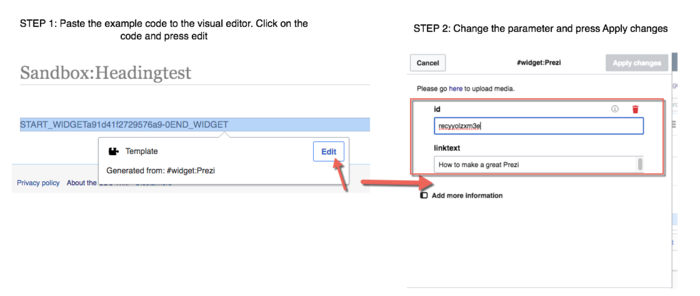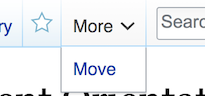# Formatting Math Text

1. To format solutions, you need to know LaTeX. The only change is that where you would normally use double dollar signs to indicate math mode, you will use html tags
 $f'(x) = \frac{1}{x^2}$
If you want to just type up your solutions in normal LaTex, you can run them through the converter on this webpage and then enter them into the wiki.
2. Because it can be tedious to be constantly typing the math html tags, if the symbols to be formatted are simple (like a single variable in the middle of a sentence), we simply italicize by using
 ''y = 0''
3. Sometimes even when you use the html math tags, the formatting just looks like italicized text. This can be particularly annoying when dealing with derivatives, for example: $f'(x)=0$. You can't really see the "prime." In order to "force" math mode, use
 $\displaystyle f'(x) = 0$
which gives $\displaystyle f'(x)=0$# LaTeX

If you've never used LaTeX before, fear not! Most of the symbols used to typeset university level mathematics are not that challenging to learn. This wikibook has a fairly comprehensive list of common symbols (you can avoid the first section on environments). However, the best way to learn the LaTeX needed to contribute to the wiki is just to look at the sample code behind certain questions. Note that since equations are never numbered, mediawiki uses \begin{align} for "\begin{align*}" and gives an error on the latter.

See the UBC wiki help pages about how to add pictures and how to upload files. The convention that we will use is to have these images have a long but similar name to the page they will be used for. Say you want to add a picture to your solution of question 1 (a) of the April 2009 exam of MATH101. The question page is at the address:

Science:Math_Exam_Resources/Courses/MATH101/April_2009/Question_1_(a)

Then the picture material for that question (for hints, questions, solutions etc.) should be all uploaded under the path:

File:Math_Exam_Resources_Courses_MATH101_April_2009_Question_1_(a)_picture_1.png

where you can change picture_1 to anything you want and the .png has to match the type of the file you are uploading.

Note that when writing the name of the file, you don't write the File: part, the upload will do it on its own, so when in the upload page, when asked for the new filename, just write:

Math_Exam_Resources_Courses_MATH101_April_2009_Question_1_(a)_picture_1.png

# Collapsible Tables

To create (basic) collapsible tables, use the following code:

{| class="collapsible wikitable collapsed" width="100%" style="background: #FFFFFF;"
! style="padding-left:1em; text-align: left;" | TITLE GOES HERE
|-
CONTENT GOES HERE
|} 

This widget allows you to add | Wolfram Alpha widgets to your wiki page.

To insert this widget, use the following code:

{{#widget:WolframAlpha|id=c44e503833b64e9f27197a484f4257c0}}


## Parameters

Note: all parameters come from the publish page of the Widget Builder.

## Sample result

#### Adding the code to the Visual editor

When you insert a widget code (including YouTube, Slideshare, etc.) to the Visual Editor, copy the code then paste it to the Visual Editor. Then click on the code, and press edit. Then, you can change the parameter and press Apply Changes.Paste the example code to the visual editor. Click on the code, and press edit. Then, change the parameter and press Apply Changes

# Deleting a Page

Simply add the text {{delete}} to the page in question. If not obvious, please explain your reason for deletion in the commit message. The page will be deleted by the UBC wiki administrator 30 days later.

Instead of deleting, consider moving the page instead. Eg when you want to rename Question 1 to Question 01.